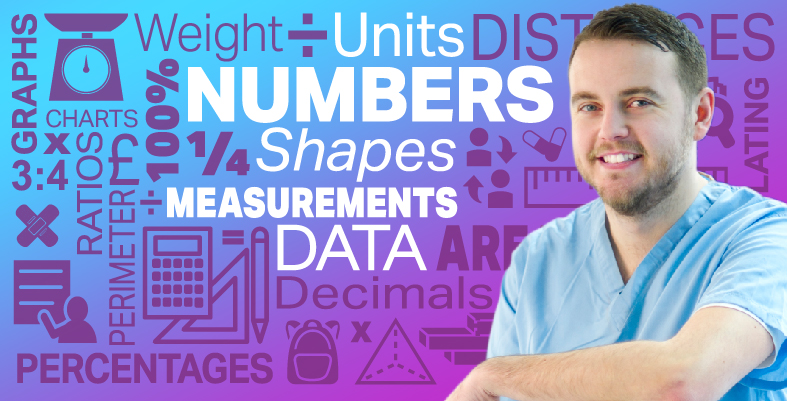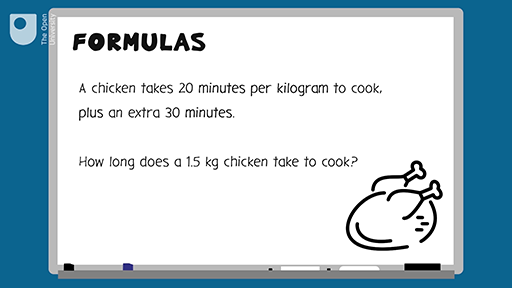Everyday maths for Health and Social Care and Education Support 1

Start this free course now. Just create an account and sign in. Enrol and complete the course for a free statement of participation or digital badge if available.

Free course

# 9.1 Formulas in words

You use formulas a lot throughout a normal day, as the examples below show.

## Example: A formula to calculate earnings

Daniel is paid £6.50 per hour. How much does he earn in ten hours?

### Method

You’re told that ‘Daniel is paid £6.50 per hour’.

This is a formula. You can use it to work out how much Daniel earns in a given number of hours. The calculation you need to do is:

Daniel’s pay = £6.50 × number of hours

You’ve been asked how much Daniel earns in ten hours, so put ‘10’ into the calculation in place of ‘number of hours’:

£6.50 × 10 = £65.00

You can use the same formula to work out how much Daniel earns for any number of hours.

You will need to be able to use formulas that have more than one step. The next example looks at a two-step formula.

## Example: A cooking formula

What are the two steps in word formulas? Watch the following video to find out.Skip transcript

#### Transcript

A chicken takes 20 minutes per kilogram to cook, plus an extra 30 minutes. So how long does a 1.5-kilogram chicken take to cook? The formula here is 20 minutes per kilogram, plus 30 minutes.

So to work out how long a 1.5-kilogram chicken takes to cook, you’ll need a formula with two steps.

Step 1: 20 minutes times number of kilograms.

So if you write this out as a formula: 20 times number of kilograms plus 30 equals cooking time. You don’t need to worry about why there are brackets in this formula. That’s how we know that we need to do this sum first before we add the 30 minutes.

Then you just need to put the numbers in the right place. In this case, you would replace number of kilograms with 1.5. 20 times 1.5 plus 30 equals 60 minutes. Your chicken will take one hour to cook.

End transcript

Interactive feature not available in single page view (see it in standard view).

Now test your learning with the following word problems.

## Activity 21: Using formulas

1. Harvey earns £7.75 per hour. How much will Harvey earn in 8 hours?

To answer this you need to multiply the amount Harvey earns in an hour (£7.75) by the number of hours (eight):

• £7.75 × 8 = £62.00
1. Use the following rule to convert a patient’s weight from stones and pounds into kilograms:
• Multiply the number of stones by 14. Add the number of pounds. Divide the answer by 2.2.
• a.Convert 8 stone 10 pounds into kilograms.
• b.Convert 12 stone 3 pounds into kilograms.

• a.You need to use a three-step formula to answer each of these questions:

• Step 1: 8 stone × 14 = 112

112 + 10 = 122

Step 3: Divide 122 by 2.2:

122 ÷ 2.2 = 51 kg (rounded to the nearest whole number).

• b.Using the same three-step formula, 12 stone 3 pounds would be:
• 8 × 14 = 168
• 168 + 3 = 171
• 171 ÷ 2.2 = 78 kg (rounded to the nearest whole number).
1. Private health insurance costs £15 a month for the first four months, then £20 a month after that. How much will the insurance cost for one year?

The information in the question gives you two formulas. To answer the question you need to find the answers to both formulas and add the results together.

The insurance costs £15 a month for the first four months. So the formula for this part of the contract is:

• £15 × 4 = £60

After the first four months the insurance is £20 a month. The question asks you the total cost of the health insurance for one year, so you need to calculate how much you would pay for another eight months:

• £20 × 8 = £160

So the total cost of the private health insurance for one year is:

• £60 + £160 = £220
FSM_SSH_1